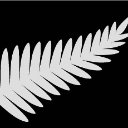## SGPLOT

``````ods close;
ods layout gridded columns=2;
ods graphics/width=15cm height=10cm;

ods listing gpath="/folders/myfolders/CMAXCI/";
ods graphics on /imagefmt=TIFF;
ods region;
TITLE1 'D Aptensio Peak 1';
PROC SGPLOT DATA=afinal1;
HISTOGRAM cmmean1 /nbins=20;
refline 7.7 10.7 13.7 / axis=x  lineattrs= (thickness=2 color=black pattern=longdash);
xaxis min=5 max=14;
run;

ods listing gpath="/folders/myfolders/CMAXCI/";
ods graphics on /imagefmt=TIFF;
ods region;
TITLE1 'D Aptensio Peak 2';
PROC SGPLOT DATA=afinal2;
HISTOGRAM cmmean2/ nbins=20;
refline 7.1 9.63 12.2/ axis=x  lineattrs= (thickness=2 color=black pattern=shortdash);
xaxis min=5 max=14;
run;
ods layout end;

``````

I found a response for multiple pdf graphs to be output and I tried to use it for Tiff output.  It ran but separate graphs were out put.

Can some one tell me how to edit the code to get a single tow column graph to be output.

Thanks

1 ACCEPTED SOLUTION

Accepted SolutionsDanH_sas
SAS Super FREQ

## Re: SGPLOT

If you merge your two data sets together, try this GTL template below and see if it works for you. If the data are related, another option might be to combine your data in a way that you can use PROC SGPANEL.

``````proc template;
define statgraph two_hist;
begingraph;
layout lattice / columndatarange=data;
cell;
Entry 'D Aptensio Peak 1' / textattrs=GraphTitleText;
layout overlay / xaxisopts=(linearopts=(viewmin=5 viewmax=14));
HISTOGRAM cmmean1 / nbins=20 binaxis=false;
referenceline x=7.7 /  lineattrs= (thickness=2 color=black pattern=longdash);
referenceline x=10.7 /  lineattrs= (thickness=2 color=black pattern=longdash);
referenceline x=13.7 /  lineattrs= (thickness=2 color=black pattern=longdash);
endlayout;
endcell;
cell;
Entry 'D Aptensio Peak 2' / textattrs=GraphTitleText;
layout overlay / xaxisopts=(linearopts=(viewmin=5 viewmax=14));
HISTOGRAM cmmean2 / nbins=20 binaxis=false;
referenceline x=7.1 /  lineattrs= (thickness=2 color=black pattern=shortdash);
referenceline x=9.63 /  lineattrs= (thickness=2 color=black pattern=shortdash);
referenceline x=12.2 /  lineattrs= (thickness=2 color=black pattern=shortdash);
endlayout;
endcell;
endlayout;
endgraph;
end;
run;

ods listing gpath="/folders/myfolders/CMAXCI/";
ods graphics on /imagefmt=TIFF;
PROC sgrender DATA=merged template=two_hist;
run;
``````

Hope this helps!

Dan

4 REPLIES 4ChrisNZ
Tourmaline | Level 20

## Re: SGPLOT

The only way I know is to use proc GPLOT or GCHART and then proc GREPLAY.

## Re: SGPLOT

Thanks for the response, but I decided to prepare the graphs in R and I
obtained what I needed. This was an old post and maybe I forgot to close it
since I didn't get a reasonable response.DanH_sas
SAS Super FREQ

## Re: SGPLOT

If you merge your two data sets together, try this GTL template below and see if it works for you. If the data are related, another option might be to combine your data in a way that you can use PROC SGPANEL.

``````proc template;
define statgraph two_hist;
begingraph;
layout lattice / columndatarange=data;
cell;
Entry 'D Aptensio Peak 1' / textattrs=GraphTitleText;
layout overlay / xaxisopts=(linearopts=(viewmin=5 viewmax=14));
HISTOGRAM cmmean1 / nbins=20 binaxis=false;
referenceline x=7.7 /  lineattrs= (thickness=2 color=black pattern=longdash);
referenceline x=10.7 /  lineattrs= (thickness=2 color=black pattern=longdash);
referenceline x=13.7 /  lineattrs= (thickness=2 color=black pattern=longdash);
endlayout;
endcell;
cell;
Entry 'D Aptensio Peak 2' / textattrs=GraphTitleText;
layout overlay / xaxisopts=(linearopts=(viewmin=5 viewmax=14));
HISTOGRAM cmmean2 / nbins=20 binaxis=false;
referenceline x=7.1 /  lineattrs= (thickness=2 color=black pattern=shortdash);
referenceline x=9.63 /  lineattrs= (thickness=2 color=black pattern=shortdash);
referenceline x=12.2 /  lineattrs= (thickness=2 color=black pattern=shortdash);
endlayout;
endcell;
endlayout;
endgraph;
end;
run;

ods listing gpath="/folders/myfolders/CMAXCI/";
ods graphics on /imagefmt=TIFF;
PROC sgrender DATA=merged template=two_hist;
run;
``````

Hope this helps!

Dan

## Re: SGPLOT

This works but this was an old post and I had decided to use R which also works well.

Discussion stats
• 4 replies
• 672 views
• 0 likes
• 3 in conversation